#### CategoryDrop-Cutter

The basic CAM-algorithm called axial tool projection, or drop-cutter, works by dropping down a cutter along the z-axis, at a chosen (x,y) location, until we make contact with the CAD model. Since the CAD model consists of triangles, drop-cutter reduces to testing cutters against the triangle vertices, edges, and facets. The most advanced of the basic cutter shapes is the BullCutter, a.k.a. Toroidal cutter, a.k.a. Filleted endmill. On the other hand the most involved of the triangle-tests is the edge test. We thus conclude that the most complex code by far in drop-cutter is the torus edge test.

The opencamlib code that implements this is spread among a few files:

• millingcutter.cpp translates/rotates the geometry into a "canonical" configuration with CL=(0,0), and the P1-P2 edge along the X-axis.
• bullcutter.cpp creates the correct ellipse, calls the ellipse-solver, returns the result
• ellipse.cpp ellipse geometry
• ellipseposition.cpp represents a point along the perimeter of the ellipse
• brent_zero.hpp has a general purpose root-finding algorithm

The special cases where we contact the flat bottom of the cutter, or the cylindrical shaft, are easy to deal with. So in the general case where we make contact with the toroid the geometry looks like this: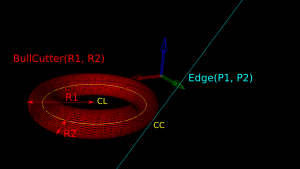Here we've fixed the XY coordinates of the cutter location (CL) point, and we're using a filleted endmill of radius R1 with a corner radius of R2. In other words R1-R2 is the major radius and R2 is the minor radius of the Torus. The edge we are testing against is defined by two points P1 and P2 (not shown). The location of these points doesn't really matter, as we do the test against an infinite line through the points (at the end we check if CC is inside the P1-P2 edge). The z-coordinate of CL is the unknown we are after, and we also want the cutter contact point (CC).

There are many ways to solve this problem, but one is based on the offset ellipse. We first realize that dropping down an R2-minor-radius Torus against a zero-radius thin line is actually equivalent to dropping down a zero-minor-radius Torus (a circle or 'ring' or CylCutter) against a R2-radius edge (the edge expanded to an R2-radius cylinder). If we now move into the 2D XY plane of this circle and slice the R2-radius cylinder we get an ellipse: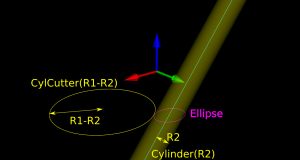The circle and ellipse share a common virtual cutter contact point (VCC). At this point the tangents of both curves match, and since the point lies on the R1-R2 circle its distance to CL is exactly R1-R2. In order to find VCC we choose a point along the perimeter of the ellipse (an ellipse-point or ePoint), find out the normal direction, and go a distance R1-R2 along the normal. We arrive at an offset-ellipse point (oePoint), and if we slide the ePoint around the ellipse, the oePoint traces out an offset ellipse.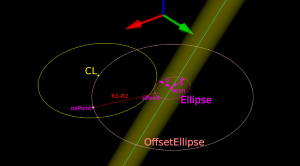Now for the shocking news: an offset-ellipse doesn't have any mathematically convenient representation that helps us solve this!
Instead we must numerically find the best ePoint such that the oePoint coincides with CL. Like so: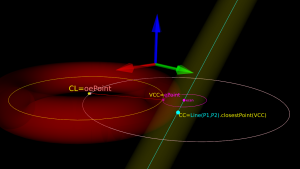Once we've found the correct ePoint, this locates the ellipse along the edge and the Z-axis - and thus CL and the cutter . If we go back to looking at the Torus it is obvious that the real CC point is now the point on the P1-P2 line that is closest to VCC.

In order to run Brent's root finding algorithm we must first bracket the root. The error we are minimizing is the difference in Y-coordinate between the oePoint and the CL point. It turns out that oePoints calculated for diangles of 0 and 3 bracket the root. Diangle 0 corresponds to the offset normal aligned with the X-axis, and diangle 3 to the offset normal  aligned with the Y-axis: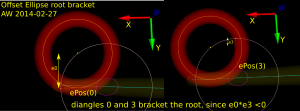Finally a clarifying drawing in 2D. The ellipse center is constrained to lie on the edge, which is aligned with the X-axis, and thus we know the Y-coordinate of the ellipse center. What we get out of the 2D computation is actually the X-coordinate of the ellipse center. In fact we get two solutions, because there are two ePoints that match our requirement that the oePoint should coincide with CL: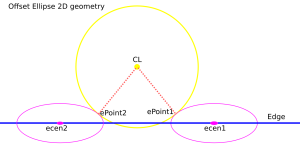Once the ellipse center is known in the XY plane, we can project onto the Edge and find the Z-coordinate. In drop-cutter we obviously approach everything from above, so between the two potential solutions we choose the one with a higher z-coordinate.

The two ePoint solutions have a geometric meaning. One corresponds to a contact between the Torus and the Edge on the underside of the Torus, while the other solution corresponds to a contact point on the topside of the Torus:

I wonder how this is done in the recently released pycam++?

Inspired by this post on the pycam forum and by this 1993 paper by Luiz Henrique de Figueiredo (or try another version) I did some work with adaptive sampling and drop-cutter today.

The point based CAM approach in drop-cutter, or axial tool-projection, or z-projection machining (whatever you want to call it) is really quite similar to sampling an unknown function. You specify some (x,y) position which you input to the drop-cutter-oracle, which will come back to you with the correct z-coordinate. The tool placed at this (x,y,z) will touch but not gouge the model. Now if we do this at a uniform (x,y) sampling rate we of course face the the usual sampling issues. It's absolutely necessary to sample the signal at a high enough sample-rate not to miss any small details. After that, you can go back and look at all pairs of consecutive points, say (start_cl, stop_cl). You then compute a mid_cl which in the xy-plane lies at the mid-point between start_cl and stop_cl and, call drop-cutter on this new point, and use some "flatness"/collinearity criterion for deciding if mid_cl should be included in the toolpath or not (deFigueiredo lists a few). Now recursively run the same test for (start_cl, mid_cl) and (mid_cl, stop_cl). If there are features in the signal (like 90-degree bends) which will never make the flatness predicate true you have to stop the subdivision/recursion at some maximum sample rate.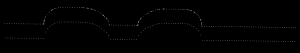Here the lower point-sequence (toolpath) is uniformly sampled every 0.08 units (this might also be called the step-forward, as opposed to the step-over, in machining lingo). The upper curve (offset for clarity) is the new adaptively sampled toolpath. It has the same minimum step-forward of 0.08 (as seen in the flat areas), but new points are inserted whenever the normalized dot-product between mid_cl-start_cl and stop_cl-mid_cl is below some threshold. That should be roughly the same as saying that the toolpath is subdivided whenever there is enough of a bend in it.

The lower figure shows a zoomed view which shows how the algorithm inserts points densely into sharp corners, until the minimum step-forward (here quite arbitrarily set to 0.0008) is reached.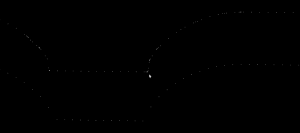If the minimum step-forward is set low enough (say 1e-5), and the post-processor rounds off to three decimals of precision when producing g-code, then this adaptive sampling could give the illusion of "perfect" or "correct" drop-cutter toolpaths even at vertical walls.

The script for drawing these pics is: http://code.google.com/p/opencamlib/source/browse/trunk/scripts/pathdropcutter_test_2.py

Here is a bigger example where, to exaggerate the issue, the initial sample-rate is very low:

I've experimented with using OpenMP to calculate drop-cutter toolpaths on a quad-core machine. These now run reasonably fast. There are obvious lurking bugs with BallCutter and BullCutter still...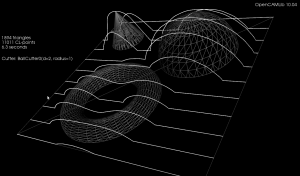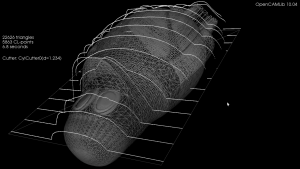Code is here: code.google.com/p/opencamlib/ (if you know C++, computational geometry, and cnc-machining, or are willing to learn, this project needs your help!)

A one-triangle test of drop-cutter for toroidal tools (a.k.a. filleted-endmills, or bull-nose).

The blue points are contacts with the facet, and the green points are contacts with the vertices. These are easy.

The edges-contacts (red-points) are a bit more involved, and are done with the offset-ellipse solver presented earlier here(the initial geometry) and here(offset-ellipse construction) and here(convergence of the solver) and here(toroid-line intesection animation).

explanation(s) to follow at some point...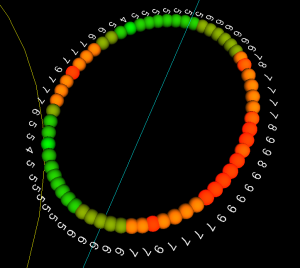In colours and numbers, the number of iterations in the offset-ellipse solver required to reach an arbitrary 8-digit precision. A smart choice of initial value can save almost half of the computation.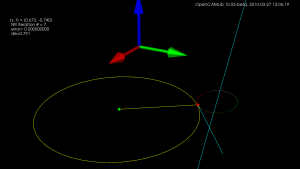More on the offset-ellipse calculation, which is related to contacting toroidal cutters against edges(lines). An ellipse aligned with the x- and y-axes, with axes a and b can be given in parametric form as (a*cos(theta) , b*sin(theta) ). The ellipse is shown as the dotted oval, in four different colours.

Now the sin() and cos() are a bit expensive the calculate every time you are running this algorithm, so we replace them with parameters (s,t) which are both in [-1,1] and constrain them so s^2 + t^2 = 1, i.e. s = cos(theta) and t=sin(theta). Points on the ellipse are calculated as (a*s, b*t).

Now we need a way of moving around our ellipse to find the one point we are seeking. At point (s,t) on the ellipse, for example the point with the red sphere in the picture, the tangent(shown as a cyan line) to the ellipse will be given by (-a*t, b*s). Instead of worrying about different quadrants in the (s,t) plane, and how the signs of s and t vary, I thought it would be simplest always to take a step in the direction of the tangent. That seems to work quite well, we update s (or t) with a new value according to the tangent, and then t (or s) is calculated from s^2+t^2=1, keeping the sign of t (or s) the same as it was before.

Now for the Newton-Rhapson search we also need a measure of the error, which in this case is the difference in the y-coordinate of the offset-ellipse point (shown as the green small sphere, and obviously calculated as the ellipse-point plus the offset-radius times a normal vector) and where we want that point. Then we just run the algorithm, always stepping either in the positive or negative direction of the tangent along the ellipse, until we reach the required precision (or max number of iterations).

Here's an animation which first shows moving around the ellipse, and then at the end a slowed-down Newton-Rhapson search which in reality converges to better than 8 decimal-places in just seven (7) iterations, but as the animation is slowed down it takes about 60-frames in the movie.

I wonder if all this should be done in Python for the general case too, where the axes of the ellipse are not parallel to the x- and y-axes, before embarking on the c++ version?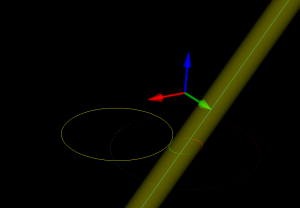Contacting a toroidal cutter (not shown) against an edge (cyan line), is equivalent to dropping down a cylindrical cutter (lower edge shown as yellow circle) against a cylinder (yellow tube) around the edge, with a radius equal to the tube-radius of the original toroidal cutter.

The plane of the tip of the cylindrical cutter slices through the yellow tube and produces an ellipse (inner green and red points). The way this example was rotated it is  obvious where the center of the ellipse along the Y-coordinate (along the green arrow) should lie. But the X-coordinate (along the red arrow) is unknown. One way of finding out is to realise that the center of the original toroidal cutter (white point) must lie on an offset-ellipse (outer green/red points). Once the X and Y coordinates are known it is fairly straightforward to find out the cutter-contact point between the cylindrical cutter and the tube, and from that the cutter-contact point between the toroid and the edge. Finally from that the cutter-location can be found.

Something to implement in opencamlib soon...

For spherical cutters (a.k.a. ball-nose), the vertex-test (green dots), and the facet-test (blue dots), are fairly trivial. The edge-test (red-dots) is slightly more involved. Here, unlike before, I tried doing it without too many calls to "expensive" functions like sin(), cos() and sqrt(). The final result of taking the maximum of all tests is shown in the "all" image which shows cutter-locations colour-coded based on the type of cutter-contact.

The logical next step is the toroidal, or bull-nose cutter. Again the edge-test is the most difficult, and I never really understood where the geometry of the offset-ellipse shows up... anyone care to explain?

After the kd-tree search is done, I've added an overlap-check which leaves only triangles with a bounding box intersecting the cutter's bounding box for the drop-cutter algorithm. It's seems like a band-aid kind of hack to get it working, I think if the tree-search would be bug free the overlap check would not be needed...

The HD-version of the video is much better, once youtube has finished processing.